## Sampling and reconstruction

CS 248 - Introduction to Computer Graphics
Autumn Quarter, 2008
Marc Levoy
Handout #6

### Table of contents: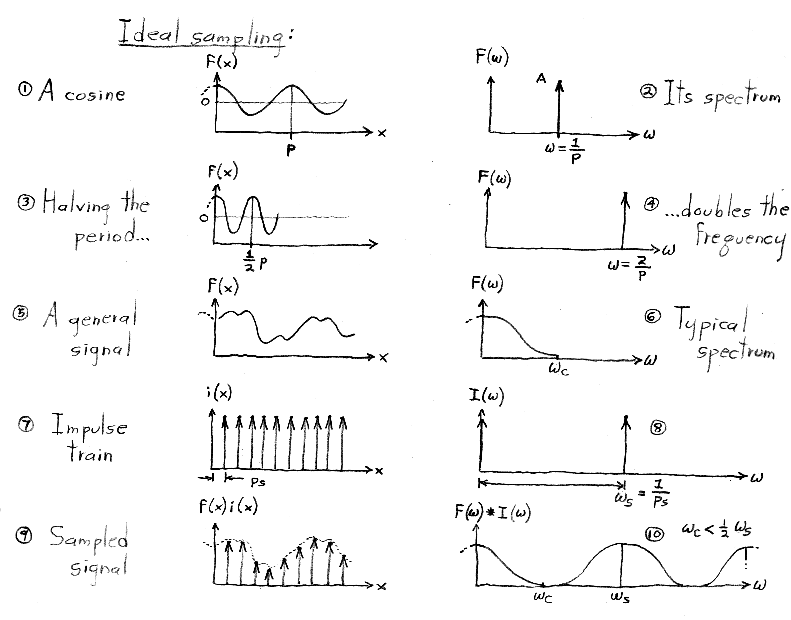Fourier analysis can be applied to any complex function that satisfies the Dirichlet conditions. However, to simplify the plots in this handout, we consider only functions that are real and even (the latter meaning symmetric about x = 0). The Fourier transform of a real and even function is also real and even. We will therefore plot only the real part of a function and its Fourier transform, and we will omit the x < 0 half of both plots. For example, the Fourier transform (plot #2) of a cosine (plot #1) consists of two impulses: one at omega = 1/p, and one at omega = -1/p, but only the first of these impulses is shown.

Although we do not manipulate impulses or impulse trains algebraically in this course, you might be wondering how they are defined. The single impulse function, dirac-delta(x), is defined as the limit of a rect function as its width goes to zero. It is drawn as a single upwards-pointing arrow of unit height located at x = 0. (The rect function, you may remember, has unit height, unit width, and is centered around x = 0.) The impulse does not have a value per se at x = 0, but is instead defined as having value = 0 for x <> 0 and integral (from negative to positive infinity) = 1. The impulse train, a.k.a. shah(x), is defined as a sum of shifted impulses. Its Fourier transform, surprisingly, is also an impulse train, where the impulses are spaced as shown above. If you're interested in more details on rects, impulses, impulse trains, and their Fourier transforms, look at the notes from CS 348B at http://graphics.stanford.edu/courses/cs348b-98/sampling/sampling1.html#Impulse Function.For proof of the surprising fact that the Fourier transform of a sinc function (plot #11) is a rect function (plot #12), look at http://graphics.stanford.edu/courses/cs348b-98/sampling/sampling1.html#Example of a transform.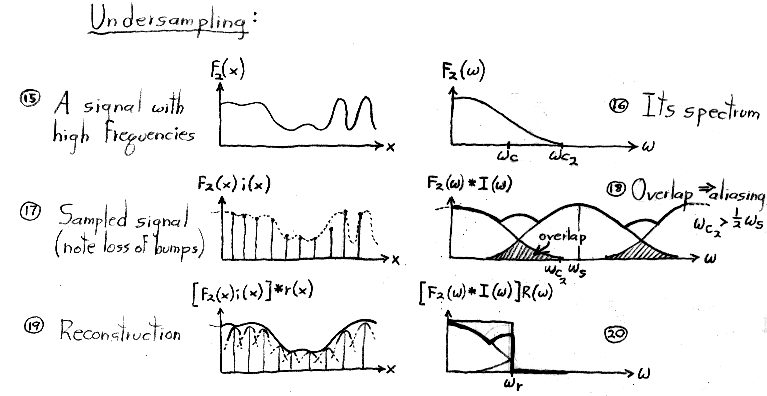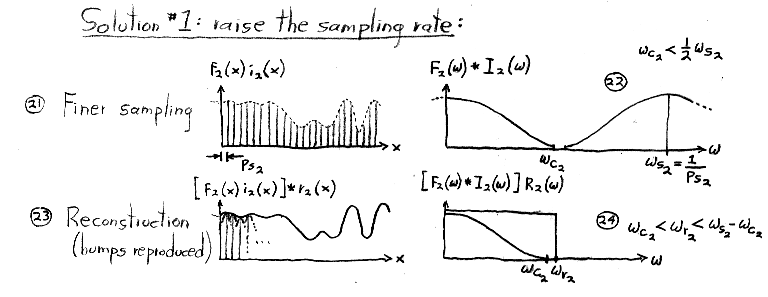Given the relationship (shown in plots #7 and #8) of the spacing between impulses in an impulse train in the spatial versus the frequency domain, it follows that decreasing their spacing in the spatial domain (plot #21) will increase their spacing in the frequency domain (plot #22). This effect can be easily proven using the similarity theorem, given at http://graphics.stanford.edu/courses/cs348b-98/sampling/sampling1.html#The similarity theorem.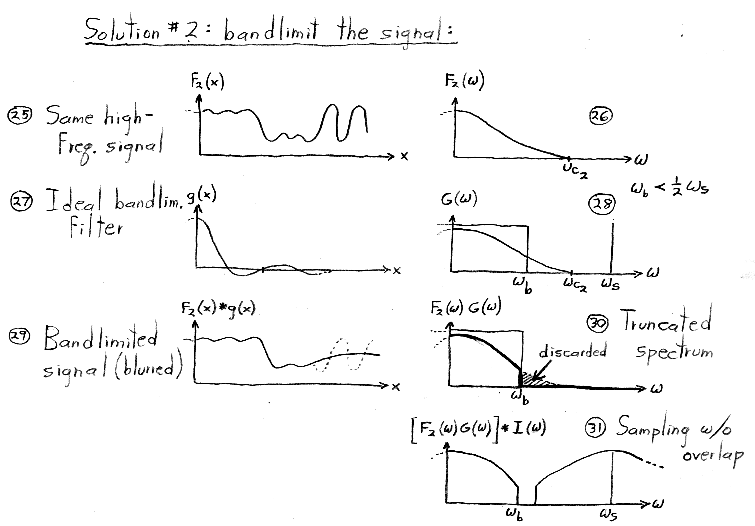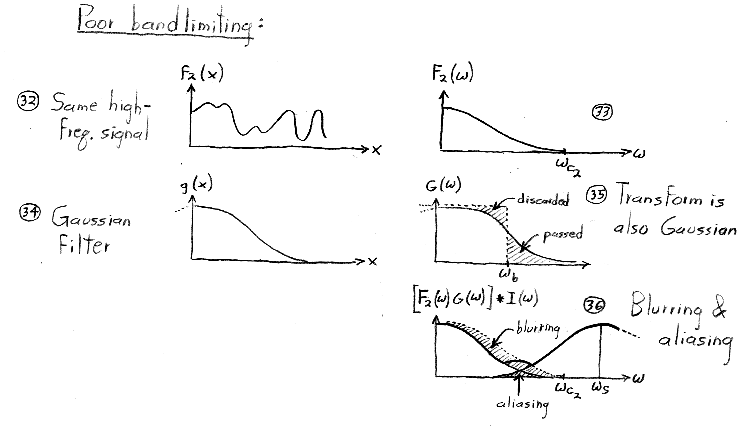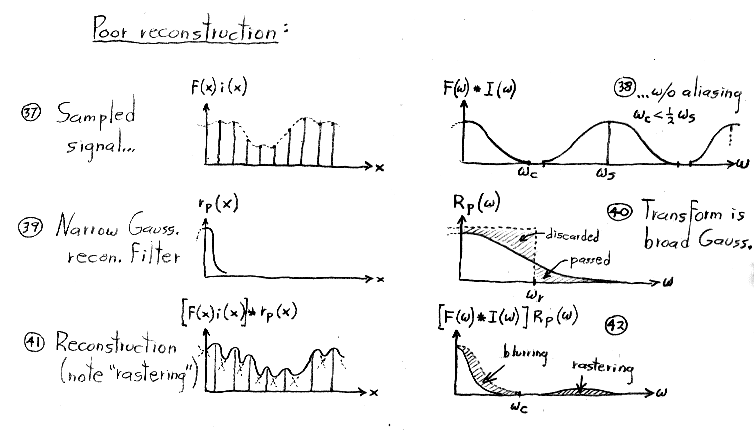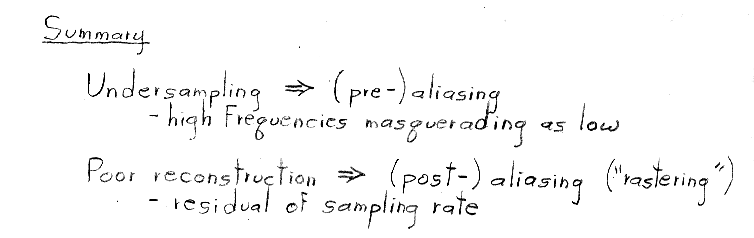levoy@cs.stanford.edu
Copyright © 2008 Marc Levoy Last update: October 1, 2008 03:49:42 PM